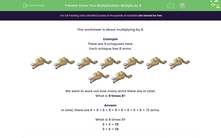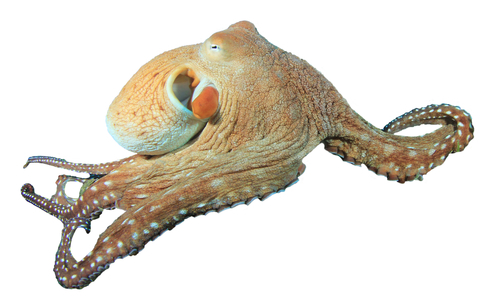# Multiply by 8, using repeated addition

In this worksheet, students multiply by 8.Key stage:  KS 2

Curriculum topic:   Number: Multiplication and Division

Curriculum subtopic:   Use Multiplication/Division Facts (3, 4 and 8)

Difficulty level:#### Worksheet Overview

In this activity, we will learn about multiplying by 8.

To multiply by eight, we can use repeated addition.

Here is an octopus. One octopus has eight arms.Let's think about what happens if we have 5 octopuses, each with 8 arms and we want to find the total number of arms!We could count the arms one by one but this would take a while!

5 groups of 8 looks like this as repeated addition

8 + 8 + 8 + 8 + 8 = 40

There are 40 arms in total!

Now let's try an example of multiplying by 8, using repeated addition.

Example

Here are 4 octopuses. They each have 8 arms. How many arms in total?In total, there are 8 + 8 + 8 + 8 = 32 arms.

What is 8 times 4?

8 × 4 = 32

4 × 8 = 32

Now that you understand repeated addition, let's try some more questions.

### What is EdPlace?

We're your National Curriculum aligned online education content provider helping each child succeed in English, maths and science from year 1 to GCSE. With an EdPlace account you’ll be able to track and measure progress, helping each child achieve their best. We build confidence and attainment by personalising each child’s learning at a level that suits them.

Get started